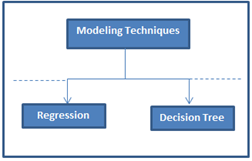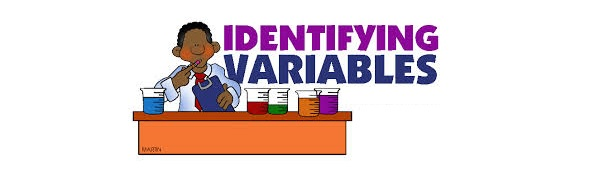## Trick to enhance power of Regression model

We, as analysts, specialize in optimization of already optimized processes. As the optimization gets finer, opportunity to make the process better gets thinner. One of the predictive modeling technique used frequently use is regression (Linear or Logistic). Another equally competing technique (typically considered as a challenger) is Decision tree.What if we could combine the benefits of both the techniques to create powerful predictive models?The trick mentioned in this article does exactly## Extracting right variables for your Regression model

Getting the right variables in your model and cleaning them can make or break your model.The precision of the model depends on the breadth (diversity) and depth (spread of data and correct transformations) of variables. This article will take you through some of the techniques used in the industry to create or transform variables. We will also cover the techniques used in the industry to select the right set of variables out of an exhaustive list created in our next article on the subject.Types## Diagnosing residual plots in linear regression models

Assumptions of Linear Regression Model :There are number of assumptions of a linear regression model. In modeling, we normally check for five of the assumptions. These are as follows :1. Relationship between the outcomes and the predictors is linear. 2. Error term has mean almost equal to zero for each value of outcome. 3. Error term has constant variance. 4. Errors are uncorrelated. 5. Errors are normally distributed or we have an adequate sample size to rely on large sample theory.The point to## 7 Types of Regression Techniques you should know!

IntroductionLinear and Logistic regressions are usually the first algorithms people learn in predictive modeling. Due to their popularity, a lot of analysts even end up thinking that they are the only form of regressions. The ones who are slightly more involved think that they are the most important amongst all forms of regression analysis.The truth is that there are innumerable forms of regressions, which can be performed. Each form has its own importance and a specific condition where they areIn the last two articles of this series (data exploration & preparation), we looked at Variable identification,Univariate, Bi-variate analysis and Missing values treatment. In this article, we will look at the next step of data preparation: Outlier detection and treatment.What is an Outlier?Outlier is a commonly used terminology by analysts and data scientists as it needs close attention else it can result in wildly wrong estimations. Simply speaking, Outlier is an observation that appears far